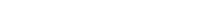# Math riddles Level 51 Answer with Hints and solutionsMath riddles game level 51 Answer with solution android game developed by Black games. Scroll Below to find details.

Math Riddles tests your IQ with mathematical puzzles. Challenge yourself with different levels of math puzzles and stretch the limits of your intelligence. Every IQ game is prepared with an approach of an IQ test. Improve your mathematics, challenge your brain with this game. There are many hard levels which you may not have solved then you can take help from here.

Math app level 51:

7,2=59

12,5=717

18,9=927

26,3=?Solution:

(7-2)(7+2)=59

(18-9)(18+9)=927

similarly

(26-3)(26+3)=2329

If there is any Doubt/discrepancy then please let me know in comments or you are unable to understand solution then I will surely help you.

### 4 thoughts on “Math Riddles App Level 51 Solution Android”

•June 12, 2019 at 5:15 pm

Ok, this is funny. You can get same result with formula

101 * 26 – 99*3 = 2329
This working for all lines except first, where
101*7 – 99*2 = 509, not 59

So at first I assumed it is a typo in game but it’s not. What a odd world are we living in.

•March 24, 2019 at 10:07 pm

¿????? (7-2)(7+2)=5×9=45. (18-9)(18+9)=9×27=243¿????????????? I don’t understand

•March 26, 2019 at 4:36 am

I’ve been trying to solve it for a week now and still I can’t wrap my head around this one

•March 28, 2019 at 5:51 pm